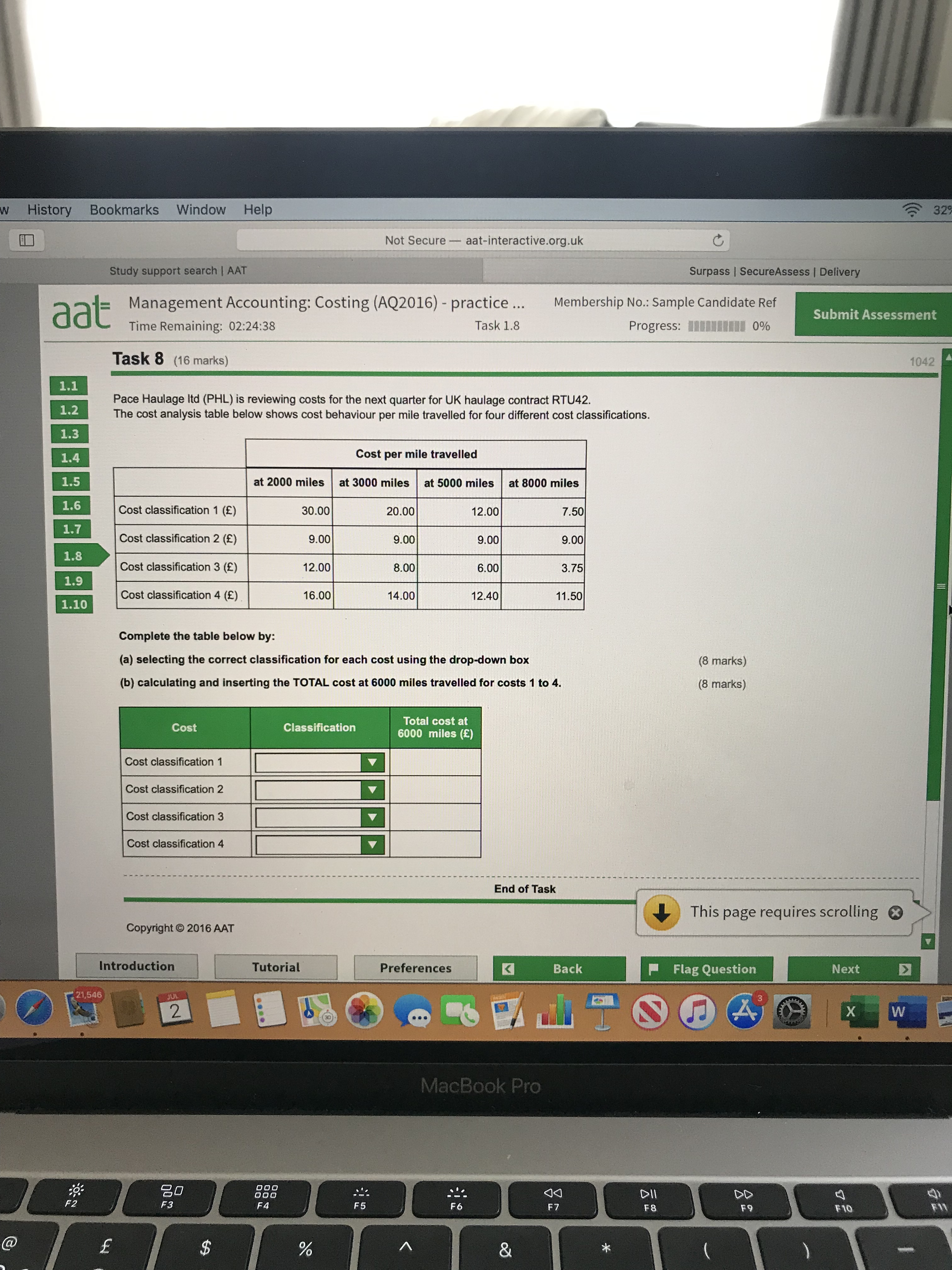• Sorry I could not add picture to previous discussion
• Work with me on this back and forth.

Cost classification 1:
@ 2000 miles it is £30 per mile = total cost £60,000
@ 3000 miles it is £20 per mile = total cost £60,000
@ 5000 miles it is £12 per mile = total cost £60,000
@ 8000 miles it is £7.5 per mile = total cost £60,000

This classification, What does it look like to you?
• Great thanks
Cost classification 1 at 6000miles =60000
Cost 2at 6000miles =54000
Cost 3 at 6000miles = 30000
Cost 4 at 6000 Miles = 72000
• Mind, you also need to say which type of classification those are.

Fixed, variable and such.
• Hello I do not understand this question, how do you calculate the total cost at 6000 miles?
• Hi
Can someone please explain calculation, how to calculate the total cost at 6000 miles?